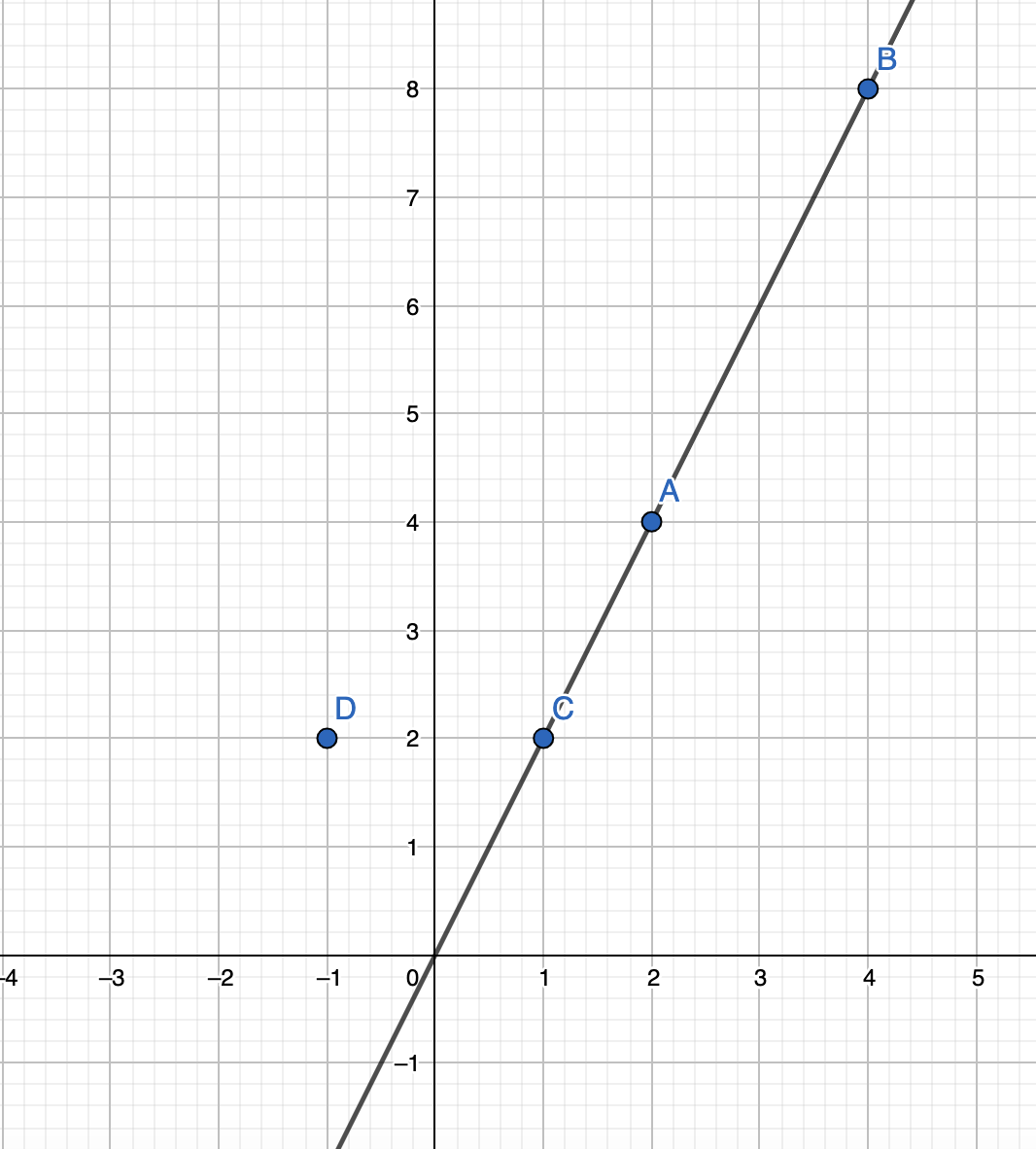# Best Line

Posted: 28 Feb, 2021
Difficulty: Easy

## PROBLEM STATEMENT

#### For example:

``````ARR = [ (2,4), (4,8), (-1,2), (1,2) ]
Here answer is 3 as (1,2), (2,4), (4,8) lie on the same line.
``````#### Note: Three points A, B, C are collinear if there exists a line that passes through all of them.

##### Input Format:
``````The first line of input contains an integer 'T' denoting the number of test cases to run. Then the test case follows.

The first line of each test case contains an integer 'N', the number of points in ARR.

The next 'N' lines of each test case contain two space-separated integers ‘X’ and ‘Y’ denoting the coordinates of the points in ARR.
``````
##### Output Format:
``````For each test case print an integer denoting the maximum number of collinear points.

Output for each test case will be printed in a separate line.
``````
##### Note:
``````You don’t need to print anything. It has already been taken care of. Just implement the given function.
``````
##### Constraints:
``````1 <= T <= 5
1 <= N <= 2000
-10^9 <= X[i], Y[i] <= 10^9

All the points in ARR are pairwise distinct.

Time Limit: 1 sec
``````Approach 1

Approach: The key observation here is that if we will fix two points on a line all other points which are collinear can be uniquely determined. We will fix a certain point and then for all the other points, we can make a counter based on the slope of the segment joining it and the fixed point.

The Algorithm will be:

1. We will store the final result in 'ANS' initialized to 1.
2. We will iterate over all the points in ‘ARR’.
• Fix this point on the line.
• Creating a counter ‘CNT’ using hashmap, to store the number of points with the same slope.
• We will now iterate over all points other than the point we have fixed-
• Calculate the slope of the segment joining it and the fixed point.
• Increment the ‘CNT’ of the slope.
• Ans is the maximum of itself and the max value of ‘CNT’.
3. Finally, return ‘ANS’.